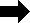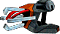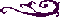OTLF FILE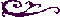Oct Tree Leaf Node Array - Level File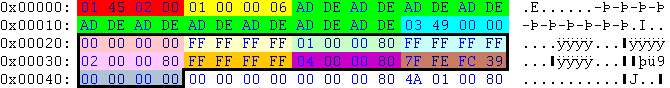HexTranslationMeaning
01 45 02 0058100581-.OTLF
01 00 00 063level 3
03 49 00 001869118691 packages follow (one package is edged in black)
Below follows the first package.
00 00 00 000, 0amount of packages (lenght); IDXA file offset *; (8 bit, 24 bit) (Thanks to Neo who figured that out.)
FF FF FF FF-1negative x neighbour
01 00 00 801, high bitpositive x neighbour
FF FF FF FF-1negative y neighbour
02 00 00 802, high bitpositive y neighbour
FF FF FF FF-1negative z neighbour
04 00 00 804, high bitpositive z neighbour
7F FE FC 39127, 127, 127, 7max. z-pos, max. y-pos, max. x-pos, cube size; (9 bit, 9 bit, 9bit, 5 bit) (Thanks to Neo who figured that out.)
00 00 00 000, 0intersected BNV count, BNV list index; (8 bit, 24 bit)

*) You have to devide the offset by 4 to get the correct offset.

A leaf node is a volume without children, because:

a) the volume is small enough, or
b) the set of polygons in the volume is small enough. (This is used in Oni. The maximum set per volume is 256 polygons.)

If the high bit is not set, the neighbour cube has children and therefore it points to the QTNA file.

Intersected gunk quads An octtree leaf (OTLF) stores its size and position and the gunk quads that intersect it. The first field contains an index/count pair that is used to access the first IDXA field that is stored in the AKOT that owns this leaf. This IDXA contains the gunk quad numbers that intersect the leaf. Intersected BNVs An octtre leaf can intersect some BNVs (see AKVA). The last field contains the index and count of this list in the second IDXA field of AKOT. Position and size The position (of leaf's positive corner) is stored as three 9 bit fields. These values are not the x, y and z coordinates themselfs but they are indexes into a constant table. This constant table has 512 entries starting with -4080.0 and going up to 4096.0 in 16 units increments. The size of leaf is stored in a 5 bit field and it is and index into another constant table. This table has 9 entries starting with 16.0 and going up to 4096.0 (each entry is double the previous one). * Maximum environment size is (8192.0, 8192.0, 8192.0) * Minimum coordinate is (-4096.0, -4096.0, -4096.0) * Maximum coordinate is (4096.0, 4096.0, 4096.0) * Minimum leaf size is 16.0 * Maximum leaf size is 4096.0 (this means that there will always be at least 8 leafs because the size of the environment is double). Neighbours A leaf can have 6 neighbours (one for each face). If a face does not have a neighbour (it's on an environment border) then the coresponding field is -1. If a neighbour field value has its high bit set then the value (without the high bit) is the index of another octtree leaf (this is the case when when the neighbour leaf is equal to or larger than the leaf). If the high bit is not set then the value is an index into a quadtree node array (the QTNA field of AKOT) (this is the case when there is more than one leaf neighbour for a face). Example The above leaf does not intersect any quads, has its maximum corner at (-2048.0, -2048.0, -2048.0), a size of 2048.0, no neighbours for all negative faces (its right in the negative corner of the environment) and positive faces have leafs as neighbours (no quadtree nodes here).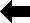Back to File Types